# Colleges with the highest SAT scores in New York

Top 10 colleges in New York with the highest SAT scores
Looking for the colleges with the highest SAT scores in New York? Well you're in luck! We've compiled a national college database and have created a list of the top 10 universities with the highest SAT scores in New York. These are the schools whose applicants had the highest average SAT scores in New York. And, since these tests are meant to determine academic prowess, they are arguably the schools with the most academically proficient students. You could even say these are the best colleges in New York. We also include each college's ACT scores and acceptance rate so that you can see where you would have the easiest or hardest time getting in. Read on to find out more.

## Columbia University in the City of New York SAT scores

The average SAT score for Columbia University in the City of New York is 1505.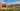The average SAT score of 1505 breaks down into:

• SAT math: 770

The average ACT score for Columbia University in the City of New York is 34 and their acceptance rate is 5.9%.

## Cornell University SAT scores

The average SAT score for Cornell University is 1480.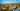The average SAT score of 1480 breaks down into:

• SAT math: 760

The average ACT score for Cornell University is 34 and their acceptance rate is 10.9%.

## Hamilton College SAT scores

The average SAT score for Hamilton College is 1440.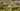The average SAT score of 1440 breaks down into:

• SAT math: 730

The average ACT score for Hamilton College is 33 and their acceptance rate is 21.3%.

## New York University SAT scores

The average SAT score for New York University is 1440.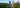The average SAT score of 1440 breaks down into:

• SAT math: 740

The average ACT score for New York University is 32 and their acceptance rate is 16.2%.

## Vassar College SAT scores

The average SAT score for Vassar College is 1440.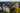The average SAT score of 1440 breaks down into:

• SAT math: 730

The average ACT score for Vassar College is 32 and their acceptance rate is 24.6%.

## University of Rochester SAT scores

The average SAT score for University of Rochester is 1420.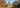The average SAT score of 1420 breaks down into:

• SAT math: 750

The average ACT score for University of Rochester is 31 and their acceptance rate is 31.4%.

## Colgate University SAT scores

The average SAT score for Colgate University is 1415.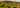The average SAT score of 1415 breaks down into:

• SAT math: 725

The average ACT score for Colgate University is 33 and their acceptance rate is 24.9%.

## The Cooper Union SAT scores

The average SAT score for The Cooper Union is 1413.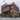Ajay Suresh / Flickr / "The Cooper Union's Foundation Building - North Side" / CC BY 2.0

The average SAT score of 1413 breaks down into:

• SAT math: 730

The average ACT score for The Cooper Union is 31 and their acceptance rate is 13.1%.

## The Jewish Theological Seminary SAT scores

The average SAT score for The Jewish Theological Seminary is 1410.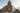The average SAT score of 1410 breaks down into:

• SAT math: 690

The average ACT score for The Jewish Theological Seminary is 29 and their acceptance rate is 59.7%.

## Rensselaer Polytechnic Institute SAT scores

The average SAT score for Rensselaer Polytechnic Institute is 1409.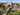The average SAT score of 1409 breaks down into:

• SAT math: 731